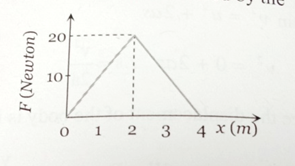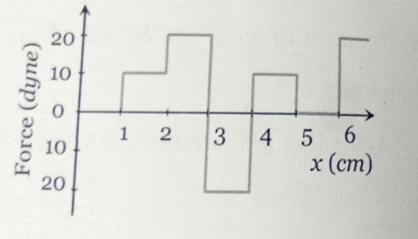Electric field is given by E $=\frac{100}{{x}^{2}}$ . Find the potential difference between x= 10 and x= 20 m. [This question is only for Dropper and XII batch]

1. 5 V

2. 10 V

3. 15 V

4. 20 V

Concept Questions :-

Integration
High Yielding Test Series + Question Bank - NEET 2020

Difficulty Level:

Calculate the electric field on the axis of a very long uniformly charged, thin rod at a distance r from one end. The charge per unit length of the rod is $\lambda$[This question is only for Dropper and XII batch]

1. $\frac{2k\lambda }{r}$

2. $\frac{k\lambda }{r}$

3. $\frac{k\lambda }{2r}$

4. $\frac{k\lambda }{4r}$

High Yielding Test Series + Question Bank - NEET 2020

Difficulty Level:

Find electric field due to a uniformly charged, long and thin rod

[This question is only for Dropper and XII batch]

1. $\frac{k\lambda }{r}$

2. $\frac{k\lambda }{2r}$

3. $\frac{2k\lambda }{r}$

4. $\frac{k\lambda }{4r}$

Concept Questions :-

Integration
High Yielding Test Series + Question Bank - NEET 2020

Difficulty Level:

A car travels 6 km towards north at an angle of 45° to the east and then travels distance of 4 km towards north at an angle of 135° to the east. How far is the point from the starting point. What angle does the straight line joining its initial and final position makes with the east?

1.

2.

3.

4.

Concept Questions :-

Resolution of vectors

Difficulty Level:

The gravitational field due to a mass distribution is given by , where k is a constant. Assuming the potential to be zero at infinity, find the potential at a point x = a. [This question is only for Dropper and XII batch]

1. $\frac{k}{{a}^{2}}$

2. $\frac{-k}{{a}^{2}}$

3. $\frac{k}{a}$

4. $\frac{-k}{a}$

Concept Questions :-

Integration
High Yielding Test Series + Question Bank - NEET 2020

Difficulty Level:

A constant force F is applied on a body. The power (P) generated is related to the time elapsed (t) as

[This question is only for Dropper and XII batch]

1.

2.

3.

4.

Concept Questions :-

Integration
High Yielding Test Series + Question Bank - NEET 2020

Difficulty Level:

A spring of spring constant  is stretched initially by 5 cm from the unstretched position. Find the work required to stretch it further by another 5 cm is   [This question is only for Dropper and XII batch]

1. 15 J

2. 18.75 N .m

3. 20 J

4. 22.75 N .m

Concept Questions :-

Integration
High Yielding Test Series + Question Bank - NEET 2020

Difficulty Level:

A long spring is stretched by 2 cm, its potential energy is U. If the spring is streched by 10 cm, find the potential energy stored in it.   [This question is only for Dropper and XII batch]

1. 10 U

2. 15 U

3. 20 U

4. 25 U

Concept Questions :-

Integration
High Yielding Test Series + Question Bank - NEET 2020

Difficulty Level:

The graph between the resistive force F acting on a body and the distance covered by the body is shown in the figure. The mass of the body is 25 kg and initial velocity is 2 m/s. When the distance covered by the body is 4 m, its kinetic energy would be    [This question is only for Dropper and XII batch]1. 50 J

2. 40 J

3. 20 J

4. 10 J

Concept Questions :-

Integration
High Yielding Test Series + Question Bank - NEET 2020

Difficulty Level:

The relationship between force and position is shown in the figure given (in one dimensional case). Find the work done by the force in displaying a body from x= 1 cm to x= 5cm is   [This question is only for Dropper and XII batch]1. 10 erg

2. 20 erg

3. 30 erg

4. 40 erg

Concept Questions :-

Integration